#### Maximum clearance

One problem that typically arises in mobile robotics is that optimal motion plans bring robots too close to obstacles. Recall from Section 6.2.4 that the shortest Euclidean paths for motion planning in a polygonal environment must be allowed to touch obstacle vertices. This motivated the maximum clearance roadmap, which was covered in Section 6.2.3. A grid-based approximate version of the maximum clearance roadmap can be made. Furthermore, a navigation function can be defined that guides the robot onto the roadmap, then travels along the roadmap, and finally deposits the robot at a specified goal. In , the resulting navigation function is called NF2.

Assume that there is a single goal state,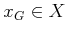. The computation of a maximum clearance navigation function proceeds as follows:

1. Instead of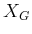, assign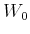to be the set of all states from which motion in at least one direction is blocked. These are the states on the boundary of the discretized collision-free space.
2. Perform wavefront iterations that propagate costs in waves outward from the obstacle boundaries.
3. As the wavefronts propagate, they will meet approximately at the location of the maximum clearance roadmap for the original, continuous problem. Mark any state at which two wavefront points arrive from opposing directions as a skeleton state. It may be the case that the wavefronts simply touch each other, rather than arriving at a common state; in this case, one of the two touching states is chosen as the skeleton state. Let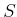denote the set of all skeleton states.
4. After the wavefront propagation ends, connect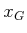to the skeleton by insertingand all states along the path to the skeleton into. This path can be found using any search algorithm.
5. Compute a navigation function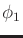overby treating all other states as if they were obstacles and using the wavefront propagation algorithm. This navigation function guides any point into the goal.
6. Treatas a goal region and compute a navigation function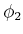using the wavefront propagation algorithm. This navigation function guides the state to the nearest point on the skeleton.
7. Combineandas follows to obtain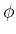. For every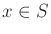, let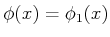. For every remaining state, the value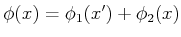is assigned, in which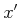is the nearest state tosuch that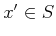. The statecan easily be recorded whileis computed.
Ifis multiply connected, then there may be multiple ways to eachby traveling around different obstacles (the paths are not homotopic). The method described above does not take into account the problem that one route may have a tighter clearance than another. The given approach only optimizes the distance traveled along the skeleton; it does not, however, maximize the nearest approach to an obstacle, if there are multiple routes.

Steven M LaValle 2020-08-14